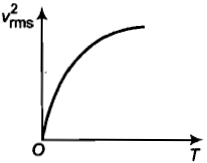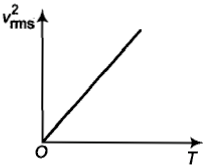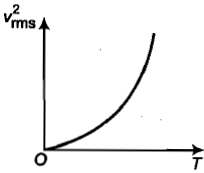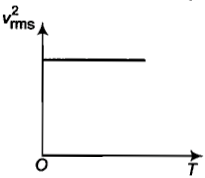You’ve reached the end of your free Videos limit.
#21-Solved-Examples-8
(Physics) > Kinetic Theory of Gases
Unable to watch the video, please try another server
Related Practice Questions :

Which of the following relations is correct for the root-mean-square speed of gas at temperature T?

Where, k= Boltzmann constant, and m= mass of a molecule.

(A)     (B)

(C)     (D)

High Yielding Test Series + Question Bank - NEET 2020

Difficulty Level:

${V}_{rms},{V}_{av},{V}_{mp}$ are root mean square, average and most probable speeds of molecules of a gas obeying Maxwellian velocity distribution. Which of the following statements is correct?

1. ${V}_{rms}<{V}_{av}<{V}_{mp}$

2. ${V}_{rms}>{V}_{av}>{V}_{mp}$

3.

4. ${V}_{avg}>{V}_{rms}<{V}_{mp}$

High Yielding Test Series + Question Bank - NEET 2020

Difficulty Level:

For a gas, the r.m.s speed at 800 K is:

(1) Four times the value at 200 K

(2) Half the value at 200 K

(3) Twice the value at 200 K

(4) Same as at 200 K

High Yielding Test Series + Question Bank - NEET 2020

Difficulty Level:

The curve between absolute temperature and ${{v}_{rms}}^{2}$ is:

(a)(b)(c)(d)High Yielding Test Series + Question Bank - NEET 2020

Difficulty Level:

Five molecules of a gas have speeds, 1, 2, 3, 4 and 5 km${s}^{-1}$.  The value of the root mean square speed of the gas molecules is:

(1) 3 km${s}^{-1}$             (2) $\sqrt{11}$km${s}^{-1}$

(3) $\frac{1}{2}\sqrt{\mathrm{\pi }}$km${s}^{-1}$    (4) 3.5 km${s}^{-1}$